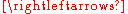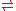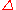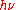xChemical equationEncyclopedia
A chemical equation is the symbolic representation of a chemical reaction
Chemical reaction
A chemical reaction is a process that leads to the transformation of one set of chemical substances to another. Chemical reactions can be either spontaneous, requiring no input of energy, or non-spontaneous, typically following the input of some type of energy, such as heat, light or electricity...

where the reactant entities are given on the left hand side and the product
Product (chemistry)
Product are formed during chemical reactions as reagents are consumed. Products have lower energy than the reagents and are produced during the reaction according to the second law of thermodynamics. The released energy comes from changes in chemical bonds between atoms in reagent molecules and...

entities on the right hand side. The coefficients next to the symbols and formulae of entities are the absolute values of the stoichiometric numbers. The first chemical equation was diagrammed by Jean Beguin
Jean Beguin
Jean Beguin was an iatrochemist noted for his 1610 Tyrocinium Chymicum , which many consider to be one of the first chemistry textbooks. In the 1615 edition of his textbook, Beguin made the first-ever chemical equation or rudimentary reaction diagrams, showing the results of reactions in which...

in 1615.

## Form

A chemical equation consists of the chemical formulas of the reactants (the starting substances) and the chemical formula of the products (substances formed in the chemical reaction). The two are separated by an arrow symbol (Arrow (symbol)
An arrow is a graphical symbol such as → or ←, used to point or indicate direction, being in its simplest form a line segment with a triangle affixed to one end, and in more complex forms a representation of an actual arrow...

, usually read as "yields") and each individual substance's chemical formula is separated from others by a plus sign.

As an example, the formula for the burning of methane
Methane
Methane is a chemical compound with the chemical formula . It is the simplest alkane, the principal component of natural gas, and probably the most abundant organic compound on earth. The relative abundance of methane makes it an attractive fuel...

can be denoted:

This equation would be read as "CH four plus O two yields CO two and H two O." But for equations involving complex chemicals, rather than reading the letter and its subscript, the chemical formulas are read using IUPAC nomenclature
IUPAC nomenclature
A chemical nomenclature is a set of rules to generate systematic names for chemical compounds. The nomenclature used most frequently worldwide is the one created and developed by the International Union of Pure and Applied Chemistry ....

. Using IUPAC nomenclature, this equation would be read as "methane plus oxygen
Oxygen
Oxygen is the element with atomic number 8 and represented by the symbol O. Its name derives from the Greek roots ὀξύς and -γενής , because at the time of naming, it was mistakenly thought that all acids required oxygen in their composition...

yields carbon dioxide
Carbon dioxide
Carbon dioxide is a naturally occurring chemical compound composed of two oxygen atoms covalently bonded to a single carbon atom...

and water."

This equation indicates that oxygen and CH4 react to form H2O and CO2. It also indicates that two oxygen molecules are required for every methane molecule and the reaction will form two water molecules and one carbon dioxide molecule for every methane and two oxygen molecules that react. The stoichiometric coefficients (the numbers in front of the chemical formulas) result from the law of conservation of mass
Conservation of mass
The law of conservation of mass, also known as the principle of mass/matter conservation, states that the mass of an isolated system will remain constant over time...

and the law of conservation of charge
Charge conservation
In physics, charge conservation is the principle that electric charge can neither be created nor destroyed. The net quantity of electric charge, the amount of positive charge minus the amount of negative charge in the universe, is always conserved...

## Common symbols

Symbols are used to differentiate between different types of reactions. To denote the type of reaction:
• "" symbol is used to denote a stoichiometric relation.
• "" symbol is used to denote a net forward reaction.
• "" symbol is used to denote a reaction in both directions.
• "" symbol is used to denote an equilibrium
Chemical equilibrium
In a chemical reaction, chemical equilibrium is the state in which the concentrations of the reactants and products have not yet changed with time. It occurs only in reversible reactions, and not in irreversible reactions. Usually, this state results when the forward reaction proceeds at the same...

.

Physical state of chemicals is also very commonly stated in parentheses after the chemical symbol, especially for ionic reactions. When stating physical state, (s) denotes a solid, (l) denotes a liquid, (g) denotes a gas and (aq) denotes an aqueous solution
Aqueous solution
An aqueous solution is a solution in which the solvent is water. It is usually shown in chemical equations by appending aq to the relevant formula, such as NaCl. The word aqueous means pertaining to, related to, similar to, or dissolved in water...

.

If the reaction requires energy, it is indicated above the arrow. A capital Greek letter delta () is put on the reaction arrow to show that energy in the form of heat is added to the reaction.is used if the energy is added in the form of light.

## Balancing chemical equations

The law of conservation of mass dictates the quantity of each element does not change in a chemical reaction. Thus, each side of the chemical equation must represent the same quantity of any particular element. Similarly, the charge is conserved in a chemical reaction. Therefore, the same charge must be present on both sides of the balanced equation.

One balances a chemical equation by changing the scalar number for each chemical formula. Simple chemical equations can be balanced by inspection, that is, by trial and error. Another technique involves solving a system of linear equations.

Ordinarily, balanced equations are written with smallest whole-number coefficients. If there is no coefficient before a chemical formula, the coefficient 1 is understood.

The method of inspection can be outlined as putting a coefficient of 1 in front of the most complex chemical formula and putting the other coefficients before everything else such that both sides of the arrows have the same number of each atom. If any fractional
Fraction (mathematics)
A fraction represents a part of a whole or, more generally, any number of equal parts. When spoken in everyday English, we specify how many parts of a certain size there are, for example, one-half, five-eighths and three-quarters.A common or "vulgar" fraction, such as 1/2, 5/8, 3/4, etc., consists...

coefficient exist, multiply every coefficient with the smallest number required to make them whole, typically the denominator of the fractional coefficient for a reaction with a single fractional coefficient.

As an example, the burning of methane would be balanced by putting a coefficient of 1 before the CH4:
Since there is one carbon on each side of the arrow, the first atom (carbon) is balanced.

Looking at the next atom (hydrogen), the right hand side has two atoms, while the left hand side has four. To balance the hydrogens, 2 goes in front of the H2O, which yields:
Inspection of the last atom to be balanced (oxygen) shows that the right hand side has four atoms, while the left hand side has two. It can be balanced by putting a 2 before O2, giving the balanced equation:
This equation does not have any coefficients in front of CH4 and CO2, since a coefficient of 1 is dropped.

## Ionic equations

An ionic equation is a chemical equation in which electrolyte
Electrolyte
In chemistry, an electrolyte is any substance containing free ions that make the substance electrically conductive. The most typical electrolyte is an ionic solution, but molten electrolytes and solid electrolytes are also possible....

s are written as dissociated ion
Ion
An ion is an atom or molecule in which the total number of electrons is not equal to the total number of protons, giving it a net positive or negative electrical charge. The name was given by physicist Michael Faraday for the substances that allow a current to pass between electrodes in a...

s. Ionic equations are used for single
Single displacement reaction
A single-displacement reaction, also called single-replacement reaction, is a type of oxidation-reduction chemical reaction when an element or ion moves out of one compound and into another. This is usually written as...This will occur if A is more reactive than B...

and double displacement reactions that occur in aqueous solution
Solution
In chemistry, a solution is a homogeneous mixture composed of only one phase. In such a mixture, a solute is dissolved in another substance, known as a solvent. The solvent does the dissolving.- Types of solutions :...

s. For example in the following precipitation reaction:
CaCl2(aq) + 2AgNO3(aq)Ca(NO3)2(aq) + 2AgCl(s)

the full ionic equation would be:
Ca2+ + 2Cl + 2Ag+ + 2NO3Ca2+ + 2NO3 + 2AgCl(s)

and the net ionic equation would be:
2Cl(aq) + 2Ag+(aq)2AgCl(s)

or, in reduced balanced form,
Ag+ + ClAgCl(s)

In this aqueous reaction the Ca2+ and the NO3 ions remain in solution and are not part of the reaction. They are termed spectator ion
Spectator ion
A spectator ion is an ion that exists as a reactant and a product in a chemical equation. Spectator ions can be observed in the reaction of aqueous solutions of sodium chloride and copper sulfate but does not affect the equilibrium:...

s and do not participate directly in the reaction, as they exist with the same oxidation state
Oxidation state
In chemistry, the oxidation state is an indicator of the degree of oxidation of an atom in a chemical compound. The formal oxidation state is the hypothetical charge that an atom would have if all bonds to atoms of different elements were 100% ionic. Oxidation states are typically represented by...

on both the reactant and product side of the chemical equation. They are only needed for charge balance of the original reagents.

In a neutralization or acid
Acid
An acid is a substance which reacts with a base. Commonly, acids can be identified as tasting sour, reacting with metals such as calcium, and bases like sodium carbonate. Aqueous acids have a pH of less than 7, where an acid of lower pH is typically stronger, and turn blue litmus paper red...

/base
Base (chemistry)
For the term in genetics, see base A base in chemistry is a substance that can accept hydrogen ions or more generally, donate electron pairs. A soluble base is referred to as an alkali if it contains and releases hydroxide ions quantitatively...

reaction, the net ionic equation will usually be:
H+ + OHH2O

There are a few acid/base reactions that produce a precipitate in addition to the water molecule shown above. An example would be the reaction of barium hydroxide
Barium hydroxide
Barium hydroxide is the chemical compound with the formula Ba2. Also known as baryta, it is one of the principal compounds of barium. The white granular monohydrate is the usual commercial form.-Preparation:...

with phosphoric acid
Phosphoric acid
Phosphoric acid, also known as orthophosphoric acid or phosphoric acid, is a mineral acid having the chemical formula H3PO4. Orthophosphoric acid molecules can combine with themselves to form a variety of compounds which are also referred to as phosphoric acids, but in a more general way...

because the insoluble salt barium phosphate is produced in addition to water.

Double displacement reactions that feature a carbonate
Carbonate
In chemistry, a carbonate is a salt of carbonic acid, characterized by the presence of the carbonate ion, . The name may also mean an ester of carbonic acid, an organic compound containing the carbonate group C2....

reacting with an acid have the net ionic equation:
2 H+ + CO32−H2O + CO2

If every ion is a "spectator ion", then there was no reaction, and the net ionic equation is null.